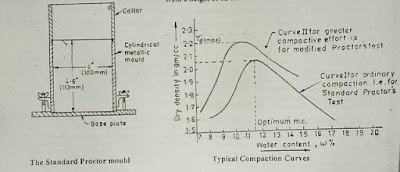Laboratory compaction test- Standard Proctor compaction test - Civil Engineering

Laboratory compaction test- Standard Proctor compaction test

The object of this test is to determine the relationship between the water content and dry density of soil and also to determine the optimum moisture content corresponding to maximum dry density.

Standard Proctor Compaction Test

Standard Proctor compaction test of soil are described below

Test Apparatus

The Apparatus used for this place consists of
1) A cylindrical metal mould having an internal diameter of 4 inches, the internal height of 4.6 inches and capacity of 1/30 cft.
2) Detachable base plate.
3) Collar.
4) Rammer having weight 5.5 Ib which is allowed to drop under gravity from a height of 12 inches.

Procedure

In performing this test, the weight of empty mould (without collar and base plate) is first of all taken. About 3 kg of soil( passing through 20 mm IS sieve) is first air-dried. It is then mixed thoroughly with a small amount of water.

This wet soil is kept covered with a wet cloth for about 5 to 30 minutes. The mould(with collar attached) is then filled with the soil in three equal layers. Each layer is compacted by 25 blows with the help of  Rammer.

After compaction of the three-layer, the soil should project slightly beyond the mould into the collar. The collar then removed and the soil is trimmed to the top of the mould. The mould containing the compacted soil is then removed from the base plate and its weight is measured. The soil is then taken out of the mould and a sample of it is then placed in an over for determining its water content(w).The Standard Proctor Mould & Typical Compaction Curve
Let, W= Weight of empty mould.
W= Weight of mould filled with a soil sample.
V = Volume of mould.
w = Water content of the soil.
Then, bulk density(𝜸t) and dry density(𝜸d)is calculated from the following relation.
𝜸 = ( W- W1 )/V
𝜸 = 𝜸/(1+w)
A number of such tests are conducted with soil sample having different moisture contents, and (𝜸d)is worked out. A graph can then be plotted between these values of water content(w) and dry density(𝜸d) and a curve are obtained as shown in the figure. The peak value of (𝜸d)can be read out from the curve which represents the optimum dry density.